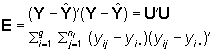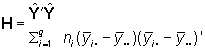# Animation of the ideas behind one-way MANOVA and HE plots

This animated series of plots illustrate the essential ideas behind the computation of hypothesis tests in a one-way MANOVA design and how these are represented by Hypothesis - Error (HE) plots.

For the multivariate linear model, Y = X B + U, hypothesis tests are based on the sums of squares and crossproducts matrices for hypothesis (H) and error (E):There are three main steps in the animation, shown by data ellipses:

1. Calculating the E matrix can be viewed as first shifting the data for each group to the centroid (grand mean) of the data.
2. Next, the separate within-group covariance matrices are averaged ("pooled") to give the E matrix.
3. Finally, the H matrix is visualized by starting with all groups centered at the grand mean, and moving finally to their positions in the data.
Use the control panel below the plot to pause or step through the animation frames.